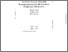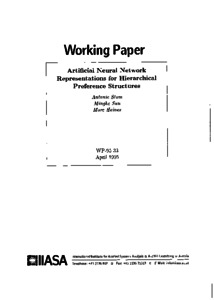# Artificial Neural Network Representations for Hierarchical Preference Structures

Stam, A., Sun, M., & Haines, M. (1995). Artificial Neural Network Representations for Hierarchical Preference Structures. IIASA Working Paper. IIASA, Laxenburg, Austria: WP-95-033Preview Text WP-95-033.pdf Download (737kB) | Preview

## Abstract

In this paper, we introduce two artificial neural network formulations that can be used to predict the preference ratings from the pairwise comparison matrices of the Analytic Hierarchy Process (AHP). First, we introduce a modified Hopfield network that can be used to exactly determine the vector of preference ratings associated with a positive reciprocal comparison matrix. The dynamics of this network are mathematically equivalent to the power method, a widely used numerical method for computing the principal eigenvectors of square matrices. However, we show that the Hopfield network representation is incapable of generalizing the preference patterns, and consequently is not suitable for approximating the preference ratings if the preference information is imprecise. Then we present a feed-forward neural network formulation that does have the ability to accurately approximate the preference ratings. A simulation experiment is used to verify the robustness of the feed-forward neural network formulation with respect to imprecise pairwise judgments. From the results of this experiment, we conclude that the feed-forward neural network formulation appears to be a powerful tool for analyzing discrete alternative multicriteria decision problems with imprecise or fuzzy ratio-scale preference judgments.

Item Type: Monograph (IIASA Working Paper) Methodology of Decision Analysis (MDA) IIASA Import 15 Jan 2016 02:06 27 Aug 2021 17:15 https://pure.iiasa.ac.at/4559View Item